Author: Oscar Cronquist Article last updated on February 07, 2023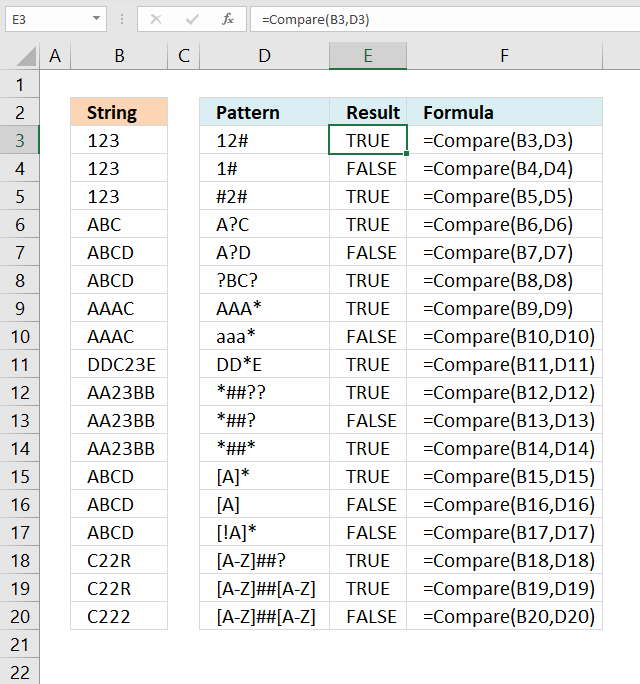The LIKE operator allows you to match a string to a pattern using Excel VBA. The image above demonstrates a macro that uses the LIKE operator to match a string to a given pattern.

The pattern is built on specific characters that I will demonstrate below.

### Excel VBA Function Syntax

result = string Like pattern

### Arguments

 result Required. Any number. string Required. A string. pattern Required. A string that meets the required pattern characters described below.

## 1. What characters can you use as a pattern in the LIKE operator?

The following characters are specifically designed to assist you in building a pattern:

 Character Desc Text ? question mark Matches any single character. * asterisk Matches zero or more characters. # number or hash sign Any single digit.

A1A* - You can also use a string combined with the characters above to build a pattern. This matches a string beginning with A1A or is equal to A1A. The asterisk matches zero characters as well.

 Characters Desc Text [abc] brackets Characters enclosed in brackets allow you to match any single character in the string. [!abc] exclamation mark The exclamation mark (!)  matches any single character not in the string. [A-Z] hyphen The hyphen lets you specify a range of characters.

### 1.1 How to make the LIKE operator case insensitive?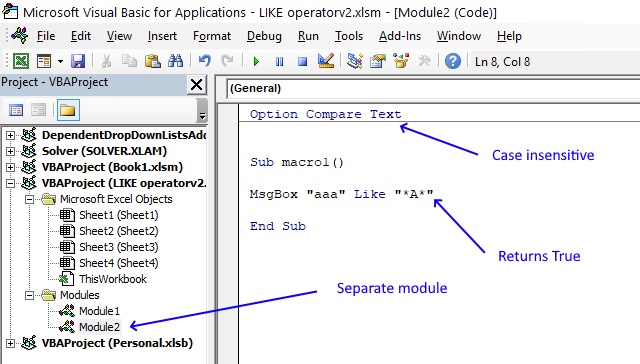Add Option compare binary or Option compare text before any macros or custom functions in your code module to change how string evaluations are made.

The default setting is Option compare binary. Use Option compare text to make the comparison case-insensitive but put the code in a separate module so other macros/functions are not affected.

 Setting Desc Option compare binary Default. Option compare text Case-insensitive evaluations.

### 1.2 What does the LIKE operator return?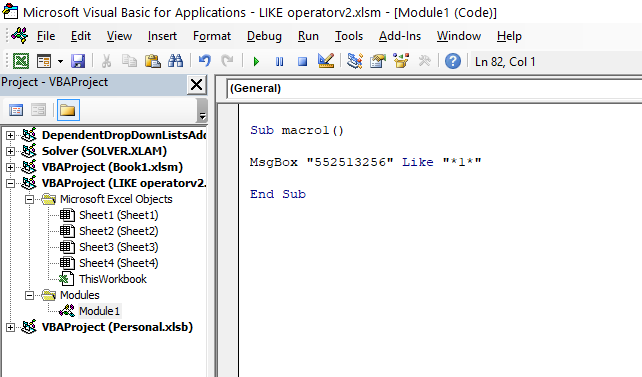The image above shows a macro in the Visual Basic Editor that returns either TRUE or FALSE depending on if the pattern matches the string or not.

Pattern *1* matches 552513256 and the macro above shows a message box containing value True.

result = string Like pattern

The LIKE operator returns a boolean value, TRUE or FALSE depending on if the pattern is a match or not. You can save the boolean value to a variable, the line above stores the boolean value in the variable result.

## 2. Compare a cell value to a pattern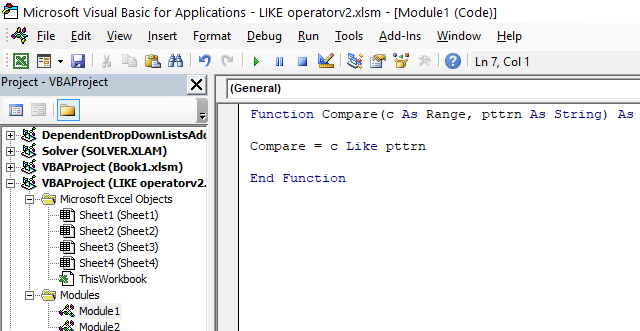This simple User Defined Function (UDF) lets you specify a pattern and compare it to a cell value. If there is a match, the function returns TRUE. If not, FALSE. I am going to use this UDF in the examples below.

```'Name User Defined Function
'Parameter c declared data type Range
'Parameter pttrn declared data type String
Function Compare(c As Range, pttrn As String) As Boolean

'Evaluate string in variable c with pattern saved to variable pttrn
'Return the result to the User Defined Function
Compare = c Like pttrn
End Function
```

Copy the code above and paste it to a code module in the VB Editor, if you want to use it. Where to put the code?

We are going to use this User Defined Function to compare patterns with strings located on a worksheet, read the next section.

### 2.1 How to use the question mark (?) character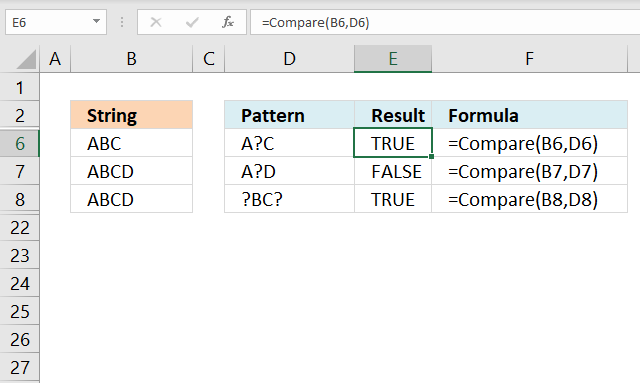The picture above demonstrates the User Defined Function we created in section 2. It takes the string in column B and compares it to the pattern in column D. A boolean value True or False is returned to column E.

UDF syntax: Compare(string, pattern)

A question mark (?) matches any single character.

Formula in cell E6:

=Compare(B6, D6)

Value in cell B6 ABC matches A?C specified in cell D6, TRUE is returned to cell E6.

Formula in cell E7:

=Compare(B7, D7)

Value in cell B7 ABCD does not match pattern A?D. BC are two characters, a question mark matches any single character. FALSE is returned in cell E3.

Formula in cell E8:

=Compare(B8, D8)

Value in cell B8 ABCD matches the pattern specified in cell D8, ?BC?. TRUE is returned to cell E8.

### 2.2 How to use the asterisk (*) character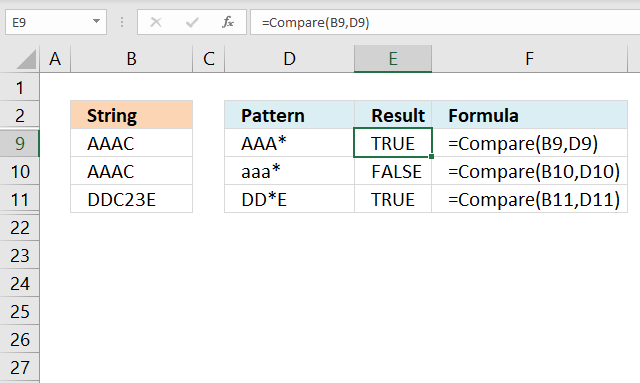The image above demonstrates the User Defined Function (UDF) described in section 2, it evaluates if a pattern matches a string using the LIKE operator. If so returns True. If not, False.

The UDF is entered in cell E9, E10, and E11. The first argument in the Compare UDF is a cell reference to the string and the second argument is a cell reference to the pattern.

Let's begin with the formula in cell E9:

=Compare(B9, D9)

The pattern tells you that the first three characters must be AAA and then the * (asterisk) matches zero or more characters. AAAC is a match to pattern AAA* and the UDF returns TRUE in cell E9.

Formula in cell E10:

=Compare(B10, D10)

aaa* does not match pattern AAAC. aaa is not equal to AAA. LIKE operator is case sensitive unless you change settings to Option Compare Text.

Formula in cell E11:

=Compare(B10, D10)

(*) matches zero or more characters, DDC23E matches DD*E.

### 2.3 How to use the number sign or hashtag (#) character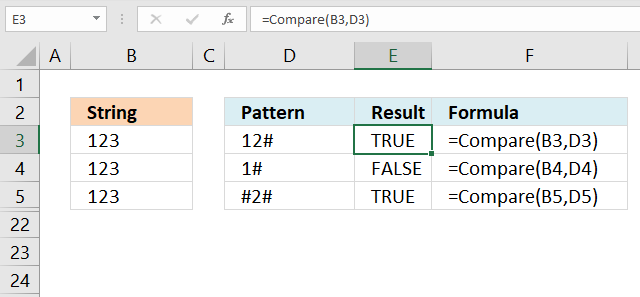The image above shows  patterns in column D and strings in column B, the hashtag character # matches a single digit. To match multiple digits use multiple #.

Formula in cell E3:

=Compare(B3, D3)

String 123 in cell B3 matches pattern 12#, TRUE is returned in cell E3.

Formula in cell E4:

=Compare(B4, D4)

String 123 in cell B4 does not match pattern 1# in cell D4, the hashtag character matches any single digit only.

Formula in cell E5:

=Compare(B5, D5)

String 123 in cell B5 matches the pattern in cell D5 #2#.

### 2.4 Combining pattern characters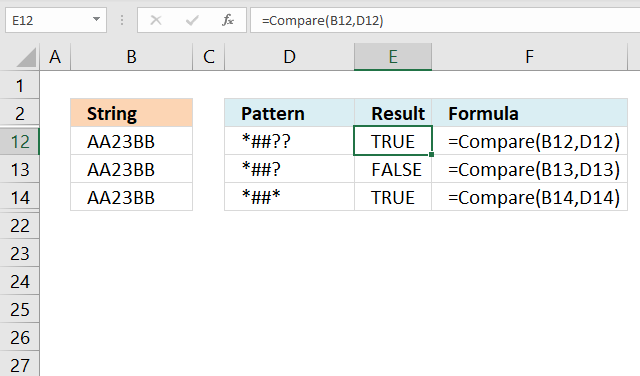The following three examples use asterisks, question marks, and number signs combined.

Formula in cell E12:

=Compare(B12, D12)

Pattern *##?? in cell D12 matches string AA23BB in cell B12, the formula returns True in cell E12. The asterisk matches 0 (zero) to any number of characters, the hashtag matches any single digit.

Note that there are two hashtags in the pattern. The ? question mark matches any single character.

Formula in cell E13:

=Compare(B13, D13)

The string in cell B13 AA23BB does not match pattern *##? specified in cell D13. There must be one character after the digits, the string has two characters after the digits.

Formula in cell E14:

=Compare(B13, D13)

The string AA23BB in cell B14 matches the pattern in cell D14 *##*. The asterisk matches 0 (zero) to any number of characters, the hashtags match two single digits and the last pattern character is the asterisk.

### 2.5 How to use brackets with the LIKE operator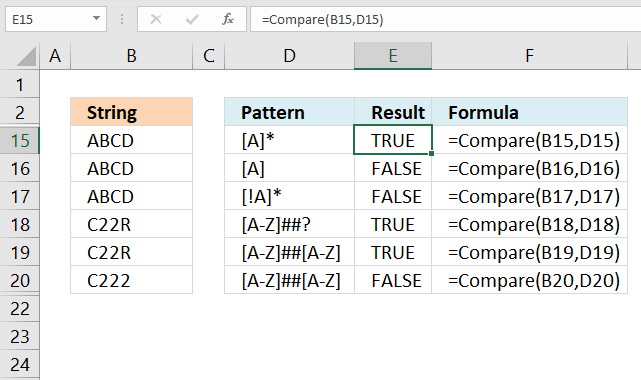Brackets match any single character you specify. A hyphen lets you compare a range of letters, however, they must be sorted from A to Z. [A-C] is a valid range but [C-A] is not valid.

Formula in cell E15:

=Compare(B15, D15)

The formula in cell E15 evaluates the string ABCD to pattern [A]* and returns TRUE. The first character in the string must be A or a, the number of remaining characters can be zero or any number in length.

Formula in cell E16:

=Compare(B16, D16)

The formula in cell E16 evaluates the string ABCD to pattern [A] and returns FALSE. The string must be only one character and that character must be A or a.

Formula in cell E17:

=Compare(B17, D17)

The formula in cell E17 compares the string ABCD to pattern [!A]* and returns FALSE. The first character in the string must be anything but character A and the number of remaining characters can be 0 (zero) or any number in length.

Formula in cell E18:

=Compare(B18, D18)

The formula in cell E18 compares the string C22R to pattern [A-Z]##? and returns TRUE. The first character in the string must be a letter between A to Z, then any two digits, and lastly a question mark that matches any single character.

Formula in cell E19:

=Compare(B19, D19)

The formula in cell E19 compares the string C22R to pattern [A-Z]##[A-Z] and returns TRUE. The string begins with any letter between A to Z, then any two digits, and lastly, any letter between A to Z.

Formula in cell E20:

=Compare(B20, D20)

The formula in cell E20 compares the string C222 to pattern [A-Z]##[A-Z] and returns FALSE. The string begins with any letter between A to Z, then any two digits, and lastly, any letter between A to Z. The string has a digit as the last character which isn't a match.

## 3. Search for a regex pattern in column and get matching values (UDF)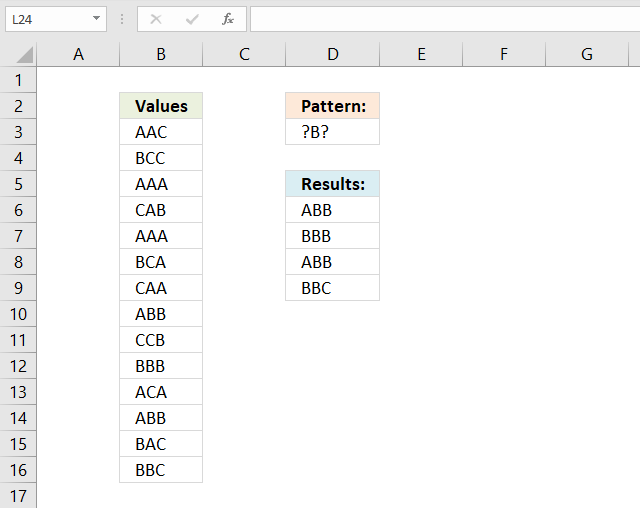The following user-defined function allows you to extract cell values using the LIKE operator and a pattern specified on the worksheet.

The formula is entered in cell D6 as an array formula, it returns multiple values to cells below if the pattern matches multiple values.

The example pattern used is in cell D3 "?B?". The question mark matches a single character, the UDF returns all values containing three letters and the middle letter is B.

Array formula in cell D6:D9:

=SearchPattern(B3:B16, D3)

How to enter an array formula

```'Name User-defined Function
Function SearchPattern(c As Range, pttrn As String)

'Dimension variables and declare data types
Dim d as String

'Iterate through each cell in c
For Each cell In c

'Check if string matches pttrn, if so concatenate cell value and comma to variable d
If cell Like pttrn Then d = d & cell & ","

'Continue with next cell
Next cell

'Split string in variable d using comma character then transpose array and return values to UDF SearchPattern
SearchPattern = Application.Transpose(Split(d, ","))
End Function
```

Where to put the code?

## 4. Search for a pattern and return values in an adjacent column (UDF)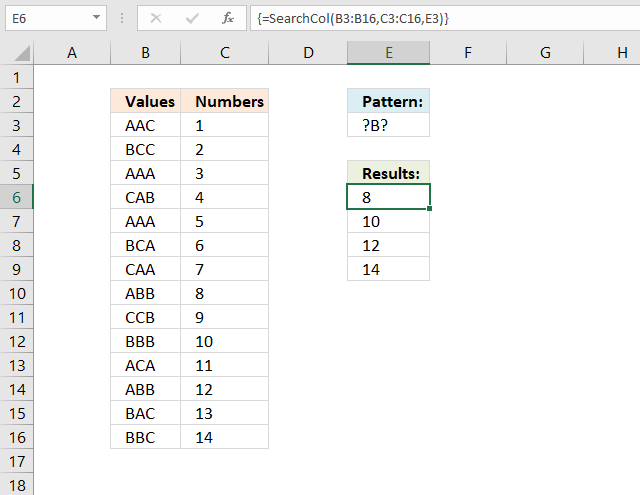This User Defined Function allows you to look for a pattern in a column and return the corresponding value in another column.

The UDF returns a value from column C if the corresponding value in column B on the same row matches the regex pattern specified in cell E3.

Array formula in cell E6:E9:

=SearchCol(B3:B16, C3:C16, E3)

How to enter an array formula

```'Name User Defined Function (UDF)
Function SearchCol(b As Range, c As Range, pttrn As String)

'Dimension variables and declare data types
Dim a As Long, d as String

'Count cells in cell range b
a = b.Cells.CountLarge

'For ... Next statement meaning iterate lines in between a times
For i = 1 To a

'Check if cell matches pattern, if so add c and a comma to variable d
If b.Cells(i) Like pttrn Then d = d & c.Cells(i) & ","
Next i

'Split values in variable d and return array to worksheet
SearchCol = Application.Transpose(Split(d, ","))
End Function
```

Where to put the code?

## 5. Extract words that match a regex pattern from cell range (UDF)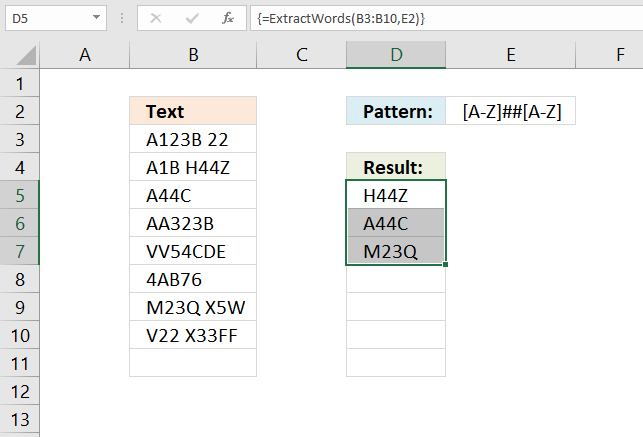The image above demonstrates a User Defined Function (UDF) that extracts words that match a given regex pattern. This means that the UDF may extract multiple words from the same cell.

You can use this UDF to extract phone numbers, zip codes, email addresses, html code, or pretty much anything from cells that contain a lot of data.

Array formula in cell D5:

=ExtractWords(B3:B10,E2)

If you want to split the data using a different character change the space character in the VBA code below to any delimiting character or characters.

### 5.1 How to enter an array formula

1. Select the cell range you want to use.
2. Press with left mouse button on in the formula bar.
3. Paste array formula to the formula bar.
4. Press and hold CTRL + SHIFT simultaneously.
5. Press Enter once.
6. Release all keys.

Your formula is now an array formula, you recognize array formulas by the beginning and ending curly brackets in the formula bar. {=array_formula}

Don't enter these characters yourself, they appear automatically.

### 5.2 VBA code

```'Name User Defined Function (UDF)
Function ExtractWords(c As Range, pttrn As String)

'Iterate through each cell in cell range c
For Each cell In c

'Split cell contents into an array using space as a delimiting character
Arr = Split(cell, " ")

'Iterate through each value in array Arr
For Each a In Arr

'Check if string in variable a matches pattern in variable pttrn
If a Like pttrn Then

'Add string a and a comma to variable d
d = d & a & ","
End If
Next

Next cell

'Split variable d using comma as a delimiting character and return array to UDF
ExtractWords = Application.Transpose(Split(d, ","))

End Function
```

## 6. Where to put the code?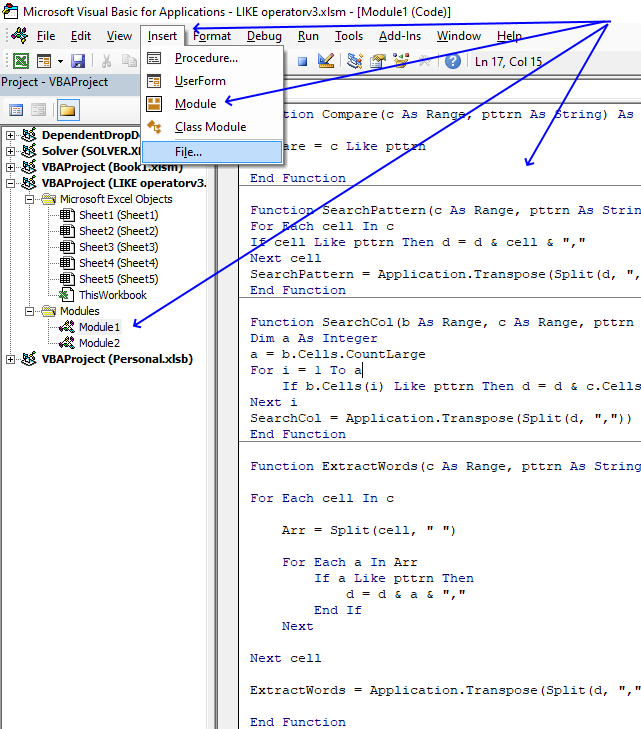1. Press Alt+F11 to open the Visual Basic Editor (VBE).
2. Press with mouse on "Insert" on the top menu, see image above.
3. A popup menu appears. Press with left mouse button on "Module" to insert a module to your workbook.
4. Copy the VBA code.
5. Paste to the code window.
Note, save your workbook with file extension *.xlsm (macro-enabled workbook) to attach the code. This step is important.

### Did you know?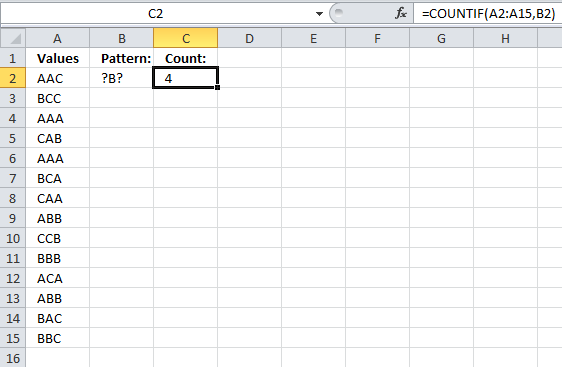You can use the question (?)  mark and asterisk (*) characters in many Excel functions. The COUNTIF function in cell C2 demonstrated in the image above counts cells in cell range A2:A15 using the pattern in cell B2.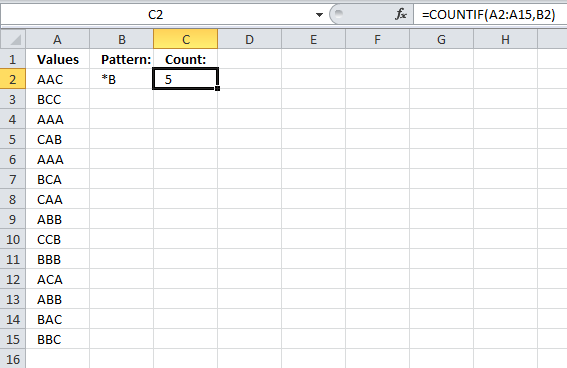If you need to use even more complicated patterns Excel allows you to use regular expressions, see this thread:
How to use Regular Expressions (Regex) in Microsoft Excel both in-cell and loops